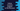# Recursion program to find the power of a number in Python## Recursion program to find the power of a number in Python:

This post will show you how to find the power of a number using a recursion function. A recursive function calls itself repeatedly until it reaches a endpoint. The endpoint is defined by a condition.

In a real python app, we can use pow() function to find the power of a number. If you don’t want to use that, you can use this approach.

We will create one separate function to do the calculation. This function will call itself repeatedly to get the result.

### Python program:

Below is the complete python program that finds the power of a number using a recursive function:

``````def find_pow(num, p):
if p == 1:
return num
else:
return find_pow(num, p - 1) * num

num = int(input("Enter the number: "))
p = int(input("Enter the power: "))

print("Result : {}".format(find_pow(num, p)))``````

Here,

• find_pow method is used to find the power of a number. It takes two arguments. The first one is the number and the second one is the power value.
• If the value of power or p is equal to 1, it returns the number num. Else, it calls the same method recursively by decrementing the value of p by 1 and multiplying that value to the number num.

If you run this program, it will print output as like below:

``````Enter the number: 3
Enter the power: 4
Result : 81``````

If you are working in a large application, you can put this function is a separate utility file and use it from any other places in your app.